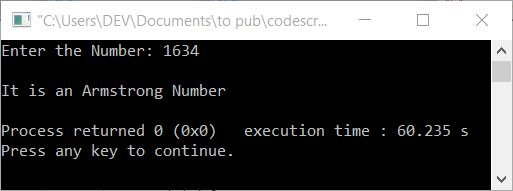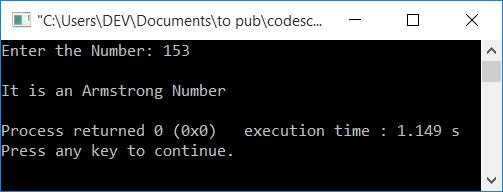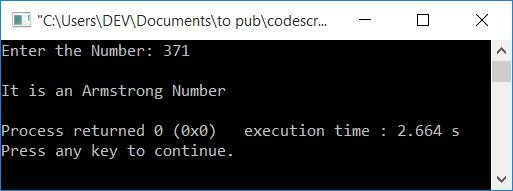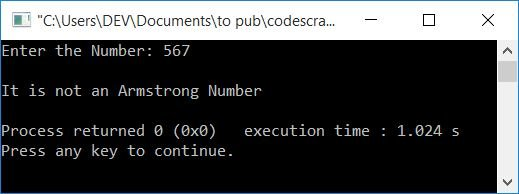# C++ program to check the Armstrong number

In this article, you will learn and get code to check whether an input number is an Armstrong number or not in C++. The program is created in these ways:

But before starting these programs, let's first understand what Armstrong's number means.

### What is an Armstrong number?

a number that equals the sum of its own digits, where each digit is raised to the power of the number of digits. For example, 1634 is an Armstrong number because:

```1634 = 14 + 64 + 34 + 44
= 1 + 1296 + 81 + 256
= 1297 + 337
= 1634```

The result is equal to the number itself. So it is an Armstrong number.

Note: Because the total number of digits in 1634 is 4, each of its digits is raised to the power of 4.

## In C++, using the while loop, check the Armstrong number

This is the first part of the article. It asks the user to enter a number and checks whether it is an Armstrong number or not.

The question is: write a program in C++ that checks whether a given number, given by the user at run-time, is an Armstrong number or not. Here is its answer:

```#include<iostream>
using namespace std;
int main()
{
intnum, temp, noOfDigit=0, res=0, rem, pow, i;
cout<<"Enter the Number: ";
cin>>num;
temp = num;
while(num>0)
{
num = num/10;
noOfDigit++;
}
num = temp;
while(num>0)
{
rem = num%10;
pow = 1;
i = 0;
while(i<noOfDigit)
{
pow = pow*rem;
i++;
}
res = res + pow;
num = num/10;
}
if(res==temp)
cout<<"\nIt is an Armstrong Number";
else
cout<<"\nIt is not an Armstrong Number";
cout<<endl;
return 0;
}```

This program was built and runs under the Code::Blocks IDE. Here is its sample run:Now supply any number, say 1634, and press the ENTER key to check whether it is an Armstrong number or not, as shown in the snapshot given below:And here is another sample run, with user input, 153:Because 153 is a three-digit number, each digit is raised to the power of three.

The following block of code:

```while(num>0)
{
num = num/10;
noOfDigit++;
}```

is used to count the total number of digits available in the given number.

The following is the dry run of the above program with user input, 153:

• Initial values, noOfDigit=0, res=0
• When user enters a number, say 153. It gets stored in num. So num=153
• Using `temp=num;`, the value of num gets initialized to temp. So temp=153
• Now the condition of the while loop gets evaluated.
• That is, the condition num>0 or 153>0 evaluates to be true, therefore program flow goes inside the loop. And num/10, 153/10, or 15 gets initialized to num. So num=15
• The value of noOfDigit is incremented. So noOfDigit=1 (because its initial value is 0).
• Program flow goes back and evaluates the condition of the while loop again.
• The process continues until its condition evaluated to be false. Before its condition is evaluated to be false, the values of num and noOfDigit will be 0 and 3. So noOfDigit=3
• Now the statement `num = temp;` initializes the value of temp to num. So num=153
• The condition of the second while loop gets evaluated. That is, the condition num>0 or 153>0 evaluates to be true, therefore program flow goes inside the loop.
• There, num%10, 153%10, or 3 gets initialized to rem. So rem=3
• and pow=1, i=0.
• Now the condition of the third while loop gets evaluated. That is, the condition i<noOfDigit or 0<3 evaluates to be true, therefore program flow goes inside the loop.
• And the pow*rem, 1*3, or 3 is initialized to pow.
• The value of i gets incremented. So i=1
• The program flow goes back and evaluates the condition, i<noOfDigit, again.
• The process continues until its condition is evaluated as false.
• Before its condition evaluated to be false, the value of pow will be 27. That is the result of 33
• So the statement, `res = res + pow;`, initializes the value of res + pow (or 0+27 or 27) to res. So res=27
• Finally, num/10 or 153/10 or 15 gets initialized to num. So num=15
• The program flow again goes back and evaluates the condition num>0 (of the while loop) again.
• The process continues until its condition is evaluated as false.
• After its condition evaluated to be false, the value of res will be 153.
• And after exiting from the loop, compare res with the original value entered by the user.
• If both are equal, then it is an Armstrong number; otherwise, it is not an Armstrong number.

## In C++, using the for loop, check the Armstrong number

Now let's create the same program as the previous one, using the for loop. That is, this program does the same job as the previous program. The only difference is that, in place of a "while" loop, we've used a "for" loop here:

```#include<iostream>
using namespace std;
int main()
{
intnum, temp, noOfDigit=0, res=0, rem, pow, i;
cout<<"Enter the Number: ";
cin>>num;
for(temp=num; temp>0; temp=temp/10)
noOfDigit++;
for(temp=num; temp>0; temp=temp/10)
{
rem = temp%10;
pow = 1;
for(i=0; i<noOfDigit; i++)
pow = pow*rem;
res = res + pow;
}
if(res==num)
cout<<"\nIt is an Armstrong Number";
else
cout<<"\nIt is not an Armstrong Number";
cout<<endl;
return 0;
}```

This program produces exactly the same output as the previous program. Here is its sample run with user input, 371:And here is the last sample run, with user input: 567.#### The same program in different languages

C++ Quiz

« Previous Program Next Program »